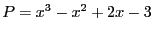Next: Second Cauchy theorem Up: First Cauchy theorem Previous: Implementation   Contents

Example

Letand the procedure:

Coeff(1)= -3;Coeff(2)=2;Coeff(3)=-1;Coeff(4)=1;
Num=Cauchy_First_Bound_Interval(3,Coeff,Bound);

Coeff_App(1)= INTERVAL(-3.1,-2.9);Coeff_App(2)=INTERVAL(1.9,2.1);
Coeff_App(3)=INTERVAL(-1.1,-0.9);Coeff_App(4)=INTERVAL(0.9,1.1);
Num=Cauchy_First_Bound_Interval(3,Coeff_App,Bound);
In the first case the procedure find that the absolute value of the roots lie in [0.6,4] while in the second case the range is [0.58,4.44444].

Jean-Pierre Merlet 2012-12-20